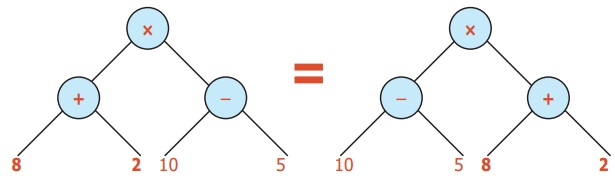Home | | Maths 6th Std | Conversion of Tree Diagrams into Numerical Expressions

# Conversion of Tree Diagrams into Numerical Expressions

When the nodes for addition and subtraction are interchanged the value remains the same which is represented using tree diagram as given below.

Conversion of Tree Diagrams into Numerical Expressions

For instance, consider the treeWe could first findas 10, thenas 5

when we multiply the results 10 and 5 we get 50. When the nodes for addition and subtraction are interchanged the value remains the same which is represented using tree diagram as given below.Does it mean that the branches also can be interchanged ? Yes, when the node is addition it is possible.But it is not possible when the node represents subtraction.Therefore from this tree diagram.The expression can be converted into either (10−5) × (8 + 2) or (8 + 2) × (10 − 5) or (2 + 8) × (10 − 5) or (10 − 5) × (2 + 8) without changing the value.

Example 11:

Convert the tree diagram into numerical expression.

Tree diagramNumerical   Expression

15− 6 + 9 .

Example 12:

Convert the Tree diagram into a numerical expression.

Tree diagramNumerical Expression

8 × 9 + 10 .

Example 13:

Convert the Tree diagram into a numerical expression.

Tree diagramNumerical Expression

10 × 6 + 6 ÷ 2 .

Example 14:

Convert the Tree diagram into a numerical expression.

Tree diagramNumerical Expression

[7 × 9 + 2] + [6 – 3 + 3].

Tags : Information Processing | Term 2 Chapter 5 | 6th Maths , 6th Maths : Term 2 Unit 5 : Information Processing
Study Material, Lecturing Notes, Assignment, Reference, Wiki description explanation, brief detail
6th Maths : Term 2 Unit 5 : Information Processing : Conversion of Tree Diagrams into Numerical Expressions | Information Processing | Term 2 Chapter 5 | 6th Maths